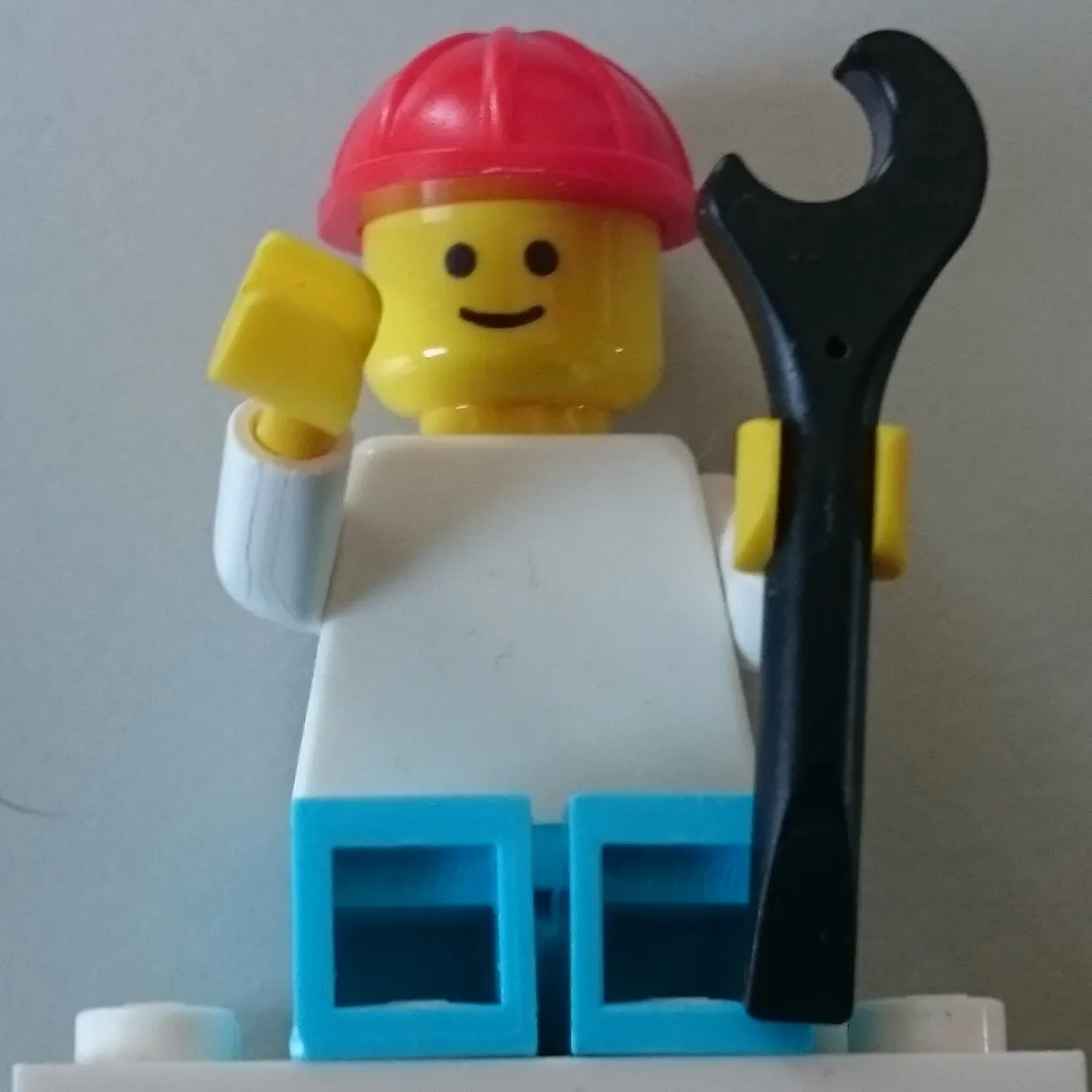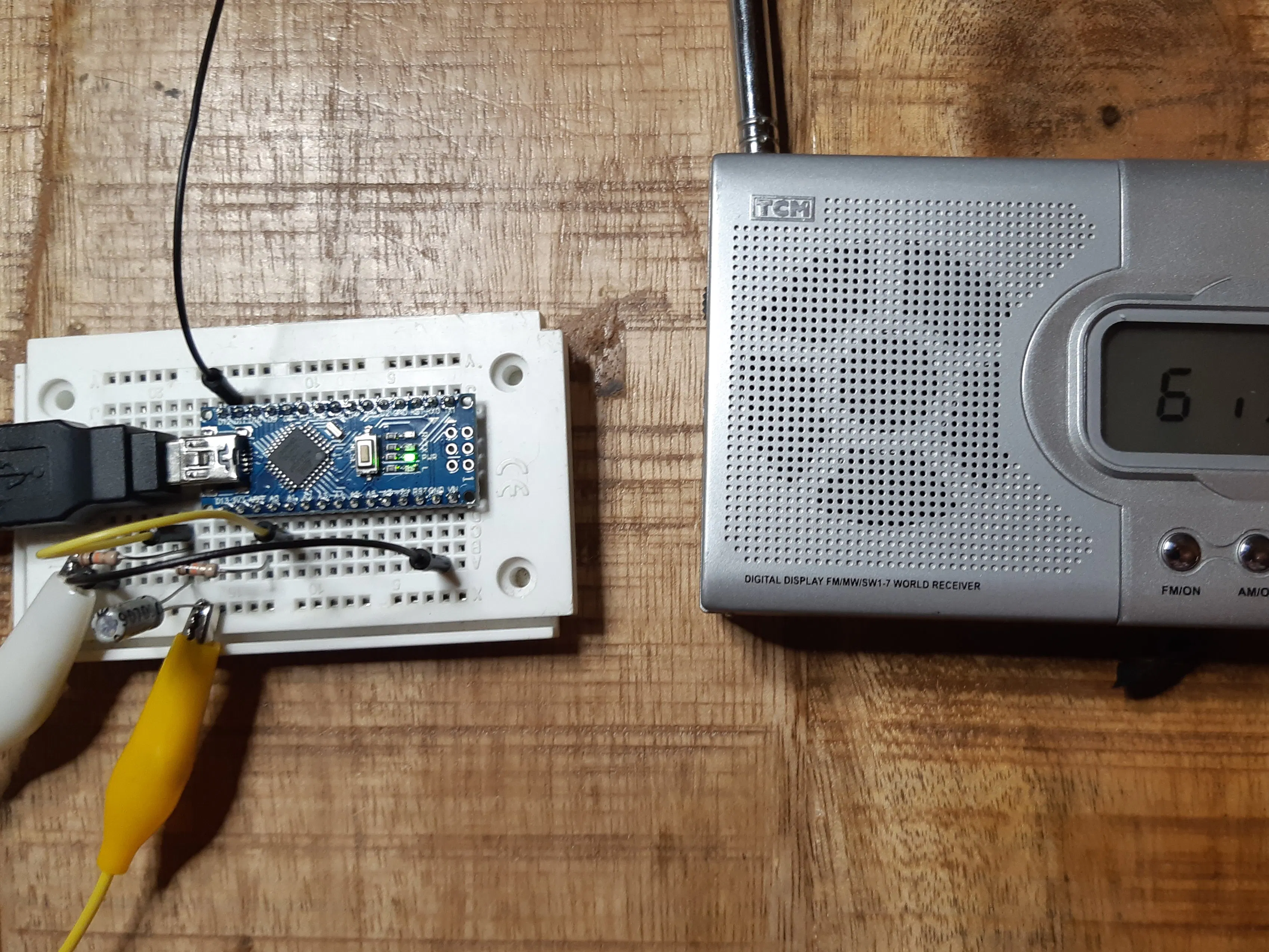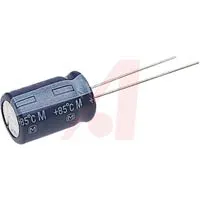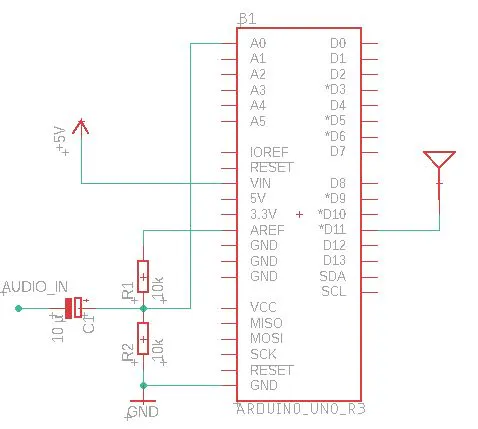# (Ab)use an Arduino as AM Music Transmitter!

See how an Arduino can be transmit music over AM with only a few lines of code.

IntermediateFull instructions provided2 hours10,462## Things used in this project

### Hardware componentsArduino Nano R3
×1Resistor 10k ohm
×2Capacitor 10 µF
×1×1

### Software apps and online servicesArduino IDE

## Schematics

### Schematic## Code

### Arduino AM Transmitter

C/C++
```/*This work is licensed under the Creative Commons Attribution-ShareAlike 4.0 International License. To view a copy of this license, visit http://creativecommons.org/licenses/by-sa/4.0/.*/

#define ANTENNA_PIN PB3 //Arduino Nano/Uno D11

// the setup function runs once when you press reset or power the board
void setup() {
Serial.begin(115200);

//Carrier Frequency generation
uint32_t fTransmit = 600; //KHz
DDRB |= (1 << ANTENNA_PIN);
TCCR2A = (0 << COM2A1) + (1 << COM2A0); //Toggle OC0A on Compare Match
TCCR2A |= (1 << WGM21) + (0 << WGM20); //CTC
TCCR2B = (0 << CS22) + (0 << CS21) + (1 << CS20); //No Prescaling
OCR2A = F_CPU / (2000 * fTransmit) - 1;

char strbuf;
sprintf(strbuf, "Will broadcast at %d KHz", (F_CPU / (2 * (1 + OCR2A)) / 1000));
Serial.println(strbuf);

//PWM Signal generation
//TCCR1A |= (0 << WGM11) + (1 << WGM10);  //Fast PWM 8 Bit
//TCCR1A |= (1 << WGM11) + (0 << WGM10);  //Fast PWM 9 Bit
TCCR1A |= (1 << WGM11) + (1 << WGM10); //Fast PWM 10 Bit
TCCR1B = (1 << WGM12);
TCCR1B |= (0 << CS12) + (0 << CS11) + (1 << CS10); //No Prescaling
TIMSK1 = (1 << OCIE1A) + (1 << TOIE1);

ADMUX = (1 << REFS1) + (1 << REFS0); //Reference internal 1.1V
}

ISR(TIMER1_OVF_vect) {
DDRB |= (1 << ANTENNA_PIN);
}

ISR(TIMER1_COMPA_vect) {
DDRB &= ~(1 << ANTENNA_PIN);
}

void loop() {
}
```